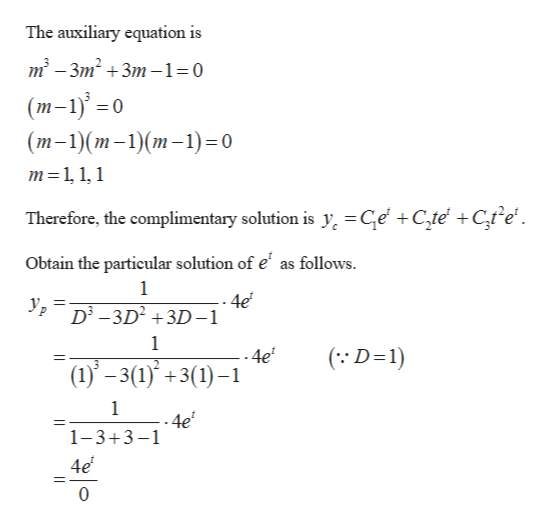# Using the method of undetermined coefficients, find the general solution to the differential equation:y'''-3y"+3y'-y=4et

Question
86 views

Using the method of undetermined coefficients, find the general solution to the differential equation:

y'''-3y"+3y'-y=4et

check_circle

Step 1

The given differential equation is y′″ - 3y″ - y = 4e                                   ...... (1)help_outlineImage TranscriptioncloseThe auxiliary equation is m2-3m2 3m-1=0 (т-1) -0 (т-1)(т-1)(т-1)-0 m 11,1 Therefore, the complimentary solution is y =Ge +C,te +Ctfe Obtain the particular solution of e' as follows. 1 4e D3-3D23D-1 1 -4e (1)-3(1)+3(1) ( D-1) 1 .4e 1-3+3-1 4e 0 fullscreen
Step 2

Now multiply the numerator with t and differentiate the denominator.

Step 3

Again multiply the numerator with t and d...

### Want to see the full answer?

See Solution

#### Want to see this answer and more?

Solutions are written by subject experts who are available 24/7. Questions are typically answered within 1 hour.*

See Solution
*Response times may vary by subject and question.
Tagged in

### Math